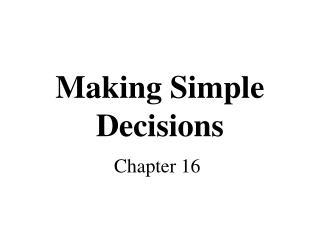DownloadDownload PresentationMaking Simple Decisions

# Making Simple Decisions

Download Presentation## Making Simple Decisions

- - - - - - - - - - - - - - - - - - - - - - - - - - - E N D - - - - - - - - - - - - - - - - - - - - - - - - - - -
##### Presentation Transcript

1. Making Simple Decisions Chapter 16

2. Topics • Decision making under uncertainty • Expected utility • Utility theory and rationality • Utility functions • Multi-attribute utility functions • Preference structures • Decision networks • Value of information

3. Uncertain Outcome of Actions • Some actions may have uncertain outcomes • Action: spend \$10 to buy a lottery which pays \$10,000 to the winner • Outcome: {win, not-win} • Each outcome is associated with some merit (utility) • Win: gain \$9990 • Not-win: lose \$10 • There is a probability distribution associated with the outcomes of this action (0.0001, 0.9999). • Should I take this action?

4. Expected Utility • Random variable X with n values x1,…,xn and distribution (p1,…,pn) • X is the outcome of performing action A (i.e., the state reached after A is taken) • Function U of X • U is a mapping from states to numerical utilities (values) • The expected utility of performing action A is EU[A] = Si=1,…,n p(xi|A)U(xi) Utility of each outcome Probability of each outcome

5. s0 A1 s1 s2 s3 0.2 0.7 0.1 100 50 70 One State/One Action Example U(A1) = 100 x 0.2 + 50 x 0.7 + 70 x 0.1 = 20 + 35 + 7 = 62

6. s0 A1 A2 s1 s2 s3 s4 0.2 0.7 0.2 0.1 0.8 100 50 70 One State/Two Actions Example • U1(A1) = 62 • U2(A2) = 74 80

7. MEU Principle • Decision theory: A rational agent should choose the action that maximizes the agent’s expected utility • Maximizing expected utility (MEU) is a normative criterion for rational choices of actions • Must have complete model of: • Actions • Utilities • States • Even if you have a complete model, will be computationally intractable

8. Decision networks • Extend Bayesian nets to handle actions and utilities • a.k.a. influence diagrams • Make use of Bayesian net inference • Useful application: Value of Information

9. Decision network representation • Chance nodes: random variables, as in Bayesian nets • Decision nodes: actions that decision maker can take • Utility/value nodes: the utility of the outcome state.

10. Airport example

11. Airport example II

12. Evaluating decision networks • Set the evidence variables for the current state. • For each possible value of the decision node (assume just one): • Set the decision node to that value. • Calculate the posterior probabilities for the parent nodes of the utility node, using BN inference. • Calculate the resulting utility for the action. • Return the action with the highest utility.

13. Exercise: Umbrella network take/don’t take P(rain) = 0.4 Umbrella Weather Lug umbrella Forecast P(lug|take) = 1.0 P(~lug|~take)=1.0 Happiness f w p(f|w) sunny rain 0.3 rainy rain 0.7 sunny no rain 0.8 rainy no rain 0.2 U(lug, rain) = -25 U(lug, ~rain) = 0 U(~lug, rain) = -100 U(~lug, ~rain) = 100

14. Value of Perfect Information (VPI) • How much is it worth to observe (with certainty) a random variable X? • Suppose the agent’s current knowledge is E. The value of the current best action  is:EU(α | E) = maxA ∑i U(Resulti(A)) p(Resulti(A) | E, Do(A)) • The value of the new best action after observing the value of X is:EU(α’ | E,X) = maxA ∑i U(Resulti(A)) p(Resulti(A) | E, X, Do(A)) • …But we don’t know the value of X yet, so we have to sum over its possible values • The value of perfect information for X is therefore: VPI(X) = ( ∑k p(xk | E)EU(αxk | xk, E)) – EU (α | E) Expected utility of the best action if we don’t know X (i.e., currently) Expected utility of the best action given that value of X Probability of each value of X

15. VPI exercise: Umbrella network What’s the value of knowing the weather forecast before leaving home? take/don’t take P(rain) = 0.4 Umbrella Weather Lug umbrella Forecast P(lug|take) = 1.0 P(~lug|~take)=1.0 Happiness f w p(f|w) sunny rain 0.3 rainy rain 0.7 sunny no rain 0.8 rainy no rain 0.2 U(lug, rain) = -25 U(lug, ~rain) = 0 U(~lug, rain) = -100 U(~lug, ~rain) = 100